# NCERT Solutions For Class 10 Maths Chapter 8 Introduction to Trigonometry

NCERT Solutions For Class 10 Maths Chapter 8 Introduction to Trigonometry

## NCERT Solutions For Class 10 Maths Chapter 8 Introduction to Trigonometry Ex 8.1

Maths Question 1.
In ∆ABC right angled at B, AB = 24 cm, BC = 7 cm. Determine:
(i) sin A, cos A
(ii) sin C, cos C
Solution:Ex 8.1 Class 10 Maths Question 2.
In given figure, find tan P – cot R.
Solution:You can also download the free PDF of Chapter 8 Ex 8.1 Introduction to Trigonometry NCERT Solutions or save the solution images and take the print out to keep it handy for your exam preparation.

Ex 8.1 Class 10 Maths Question 3.
If sin A = 3/4 , calculate cos A and tan A.
Solution:Ex 8.1 Class 10 Maths Question 4.
Given 15 cot A = 8, find sin A and sec A.
Solution:Ex 8.1 Class 10 Maths Question 5.
Given sec θ = 13/12 , calculate all other trigonometric ratios.
Solution:Ex 8.1 Class 10 Maths Question 6.
If ∠A and ∠B are acute angles such that cos A = cos B, then show that ∠A = ∠B.
Solution:Ex 8.1 Class 10 Maths Question 7.
If cot θ = 78, evaluate:
(i) (1+sinθ)(1sinθ)/(1+cosθ)(1cosθ)
(ii) cot²θ
Solution:Ex 8.1 Class 10 Maths Question 8.
If 3 cot A = 4, check whether 1tan2A/1+tan2A = cos² A – sin² A or not.
Solution: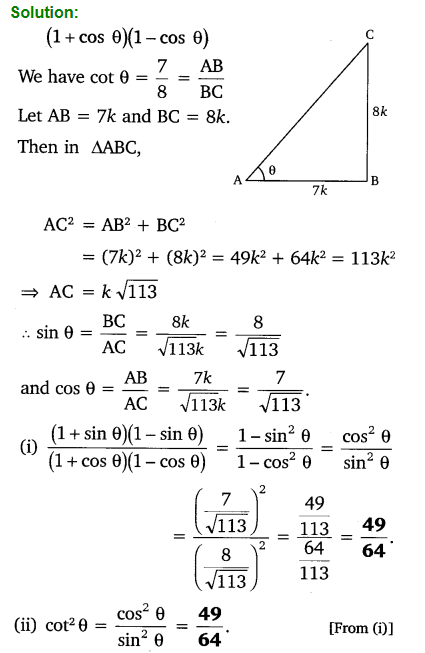Ex 8.1 Class 10 Maths Question 9.
In triangle ABC, right angled at B, if tan A = 1/3, find the value of:
(i) sin A cos C + cos A sin C
(ii) cos A cos C – sin A sin C
Solution: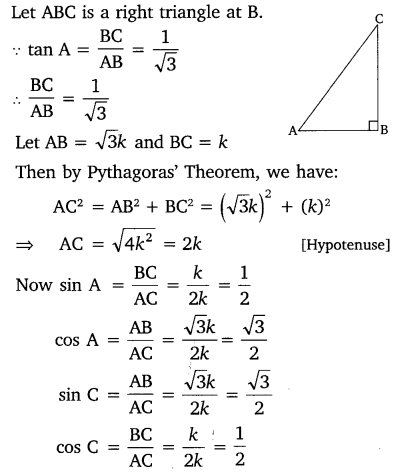Ex 8.1 Class 10 Maths Question 10.
In ΔPQR, right-angled at Q, PR + QR = 25 cm and PQ = 5 cm. Determine the values of sin P, cos P and tan P.
Solution: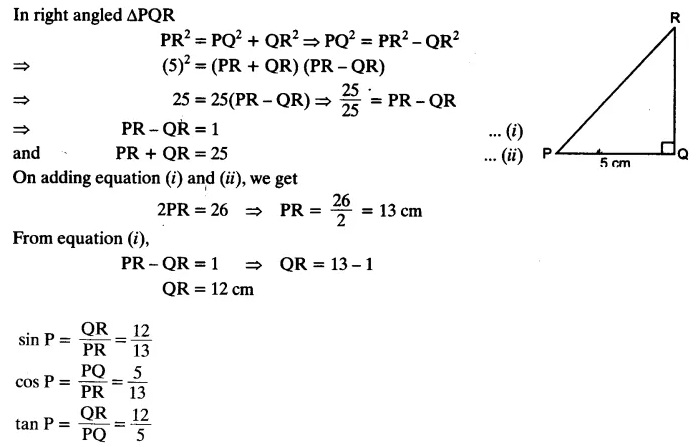Ex 8.1 Class 10 Maths Question 11.
State whether the following statements are true or false. Justify your answer.
(i) The value of tan A is always less than 1.
(ii) sec A = 12/5 for some value of angle A.
(iii) cos A is the abbreviation used for the cosecant of angle A.
(iv) cot A is the product of cot and A.
(v) sin θ = 4/3 for some angle.
Solution:### Class 10 Maths Introduction To Trigonometry

#### Trigonometry

Trigonometry is the study of relationships between the sides and angles of a right-angled triangle.

#### Trigonometric Ratios

Trigonometric ratios of an acute angle in a right triangle express the relationship between the angle and the length of its sides.
Let ∆ABC be a triangle right angled at B. Then the trigonometric ratios of the angle A in right ∆ABC are defined as follows: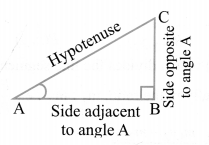Note:
The values of the trigonometric ratios of an angle do not vary with the lengths of the sides of the triangle, if the angle remains same.#### Trigonometric Ratios for Complementary Angles

sin (90° – A) = cos A
cos (90° – A) = sin A
tan (90° – A) = cot A
cot (90° – A) = tan A
sec (90° – A) = cosec A
cosec (90° – A) = sec A
Note:
Here (90° – A) is the complementary angle of A.

#### Trigonometric Identities

An equation involving trigonometric ratios of an angle is called a trigonometric identity, if it is true for all values of the angle(s) involved.
(i) sin2θ + cos2θ = 1 [for 0° ≤ θ ≤ 90°]
(ii) sec2θ – tan2θ = 1 [for 0° ≤ θ ≤ 90°]
(iii) cosec2θ – cot2θ = 1 [for 0° < θ ≤ 90°]

## NCERT Solutions for Class 10 Maths Chapter 8 Introduction to Trigonometry Ex 8.2

Class 10 Ex 8.2 Question 1.
Evaluate the following: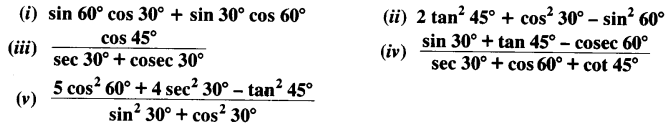Solution: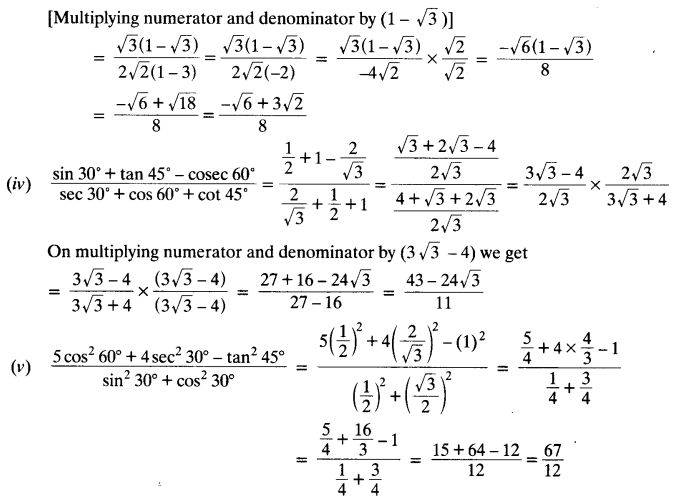Ex 8.2 Class 10 Question 2.
Choose the correct option and justify your choice:Solution: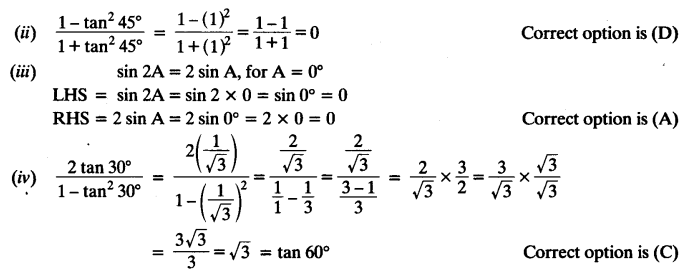Exercise 8.2 Class 10 Question 3.
If tan (A + B) = √3 and tan (A – B) = 1/3; 0° < A + B ≤ 90°; A > B, find A and B.
Solution:
tan (A + B) = √3
⇒ tan (A + B) = tan 60°
⇒ A + B = 60° ……(i)
tan (A – B) = 1/3
⇒ tan (A – B) = tan 30°
⇒ A – B = 30° ……..(ii)
Adding equation (i) and (ii), we get
2A = 90° ⇒ A = 45°
From (i), 45° + B = 60° ⇒ B = 60° – 45° = 15°
Hence, ∠A = 45°, ∠B = 15°

Exercise 8.2 Class 10 NCERT Solution Question 4.
State whether the following statements are true or false. Justify your answer.
(i) sin (A + B) = sin A + sin B.
(ii) The value of sin θ increases as θ increases.
(iii) The value of cos θ increases as θ increases.
(iv) sin θ = cos θ for all values of θ.
(v) cot A is not defined for A = 0°.
Solution:## NCERT Solutions for Class 10 Maths Chapter 8 Introduction to Trigonometry Ex 8.3

Question 1.Solution:Question 2.
Show that:
(i) tan 48° tan 23° tan 42° tan 67° = 1
(ii) cos 38° cos 52° – sin 38° sin 52° = 0
Solution:
(i) LHS = tan 48° tan 23° tan 42° tan 67°
= tan 48° tan 23° tan (90° – 48°) tan (90° – 23°)
= tan 48° tan 23° cot 48° cot 23° = tan 48° tan 23° .1/tan481/tan23
= 1 = RHS

(ii) LHS = cos 38° cos 52° – sin 38° sin 52°
= cos 38° cos (90° – 38°) – sin 38° sin (90° – 38°)
= cos 38° sin 38°- sin 38° cos 38° = 0 = RHS

Question 3.
If tan 2A = cot (A – 18°), where 2A is an acute angle, find the value of A.
Solution:
tan 2A = cot (A – 18°)
⇒ cot (90° – 2A) = cot (A – 18°) [∵cot (90° – θ) = tan θ]
⇒ 90° – 2A = A – 18° ⇒ 3A = 108° ⇒ A = 108°/3
∴ ∠ A = 36°

Question 4.
If tan A = cot B, prove that A + B = 90°.
Solution:
tan A = cot B ⇒ tan A = tan (90° – B) [ ∵ tan (90° – θ) = cot θ]
⇒ A = 90° – B ⇒ A + B = 90° Proved

Question 5.
If sec 4A = cosec (A – 20°), where 4A is an acute angle, find the value of A.
Solution:
sec 4A = cosec (A – 20°)
⇒ cosec (90° – 4A) = cosec (A – 20°) [cosec (90° – θ) = sec θ]
⇒ 90° – 4A = A – 20° ⇒ 5A = 110°
A = 110°/5
A = 22°
∴ ∠ A = 22°

Question 6.
If A, Band Care interior angles of a triangle ABC, then show that: sin (B+C/2) = cos A/2
Solution: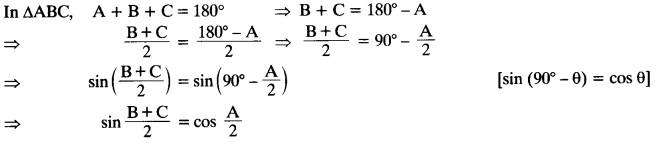Question 7.
Express sin 61° + cos 75° in terms of trigonometric ratios of angles between 0° and 45°.
Solution:
sin 67° + cos 75° = sin (90° – 23°) + cos (90° – 15°) = cos 23° + sin 15°

## NCERT Solutions for Class 10 Maths Chapter 8 Introduction to Trigonometry Ex 8.4

Question 1.
Express the trigonometric ratios sin A, sec A and tan A in terms of cot A.
Solution:
From trigonometric identity, cosec² A – cot² A = 1, we getQuestion 2.
Write all the other trigonometric ratios of ∠A in terms of sec A.
Solution:
Since sin² A + cos² A = 1, thereforeQuestion 3.
Evaluate:
(i)(ii) sin 25° cos 65° + cos 25° sin 65°
Solution: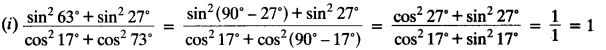(ii) sin 25° cos 65° + cos 25° sin 65° = sin 25° cos (90° – 25°) + cos 25° sin (90° – 25°)
= sin 25° sin 25° + cos 25° cos 25°
= sin² 25° + cos² 25° = 1

Question 4.
Choose the correct option. Justify your choice.
(i) 9 sec² A – 9 tan² A =
(A) 1
(B) 9
(C) 8
(D) 0

(ii) (1 + tan θ + sec θ) (1 + cot θ – cosec θ ) =
(A) 0
(B) 1
(C) 2
(D) -1

(iii) (sec A + tan A) (1 – sin A) =
(A) sec A
(B) sin A
(C) cosec A
(D) cos A

(iv) 1+tan2A/1+cot2A
(A) sec² A
(B) -1
(C) cot² A
(D) tan² A
Solution:
(i) 9 sec² A – 9 tan² A = 9(sec² A – tan² A) = 9 x 1 = 9
Correct option is (B)Question 5.
Prove the following identities, where the angles involved are acute angles for which the expressions are defined.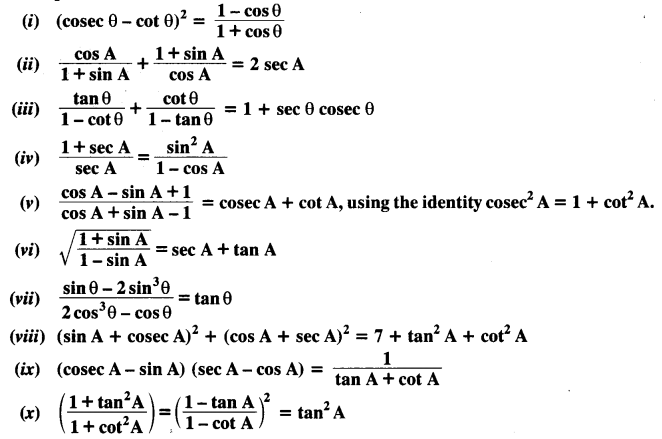Solution: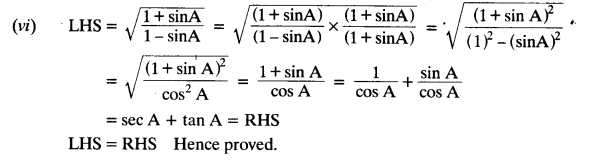## Introduction to Trigonometry Class 10 Extra Questions Maths Chapter 8## SabDekho

The Complete Educational Website